Next: Elliptic orbits Up: Keplerian orbits Previous: Orbital energies

# Transfer orbits

Consider an artificial satellite in an elliptical orbit around the Sun (the same considerations also apply to satellites in orbit around the Earth). At perihelion,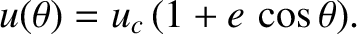, and Equations (4.41) and (4.44) can be combined to give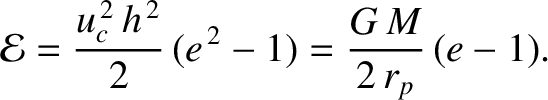(4.46)

Here,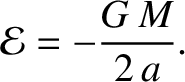is the satellite's tangential velocity, and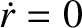is the tangential velocity that it would need to maintain a circular orbit at the perihelion distance. Likewise, at aphelion,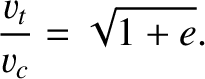(4.47)

where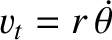is now the tangential velocity that the satellite would need to maintain a circular orbit at the aphelion distance.

Suppose that our satellite is initially in a circular orbit of radius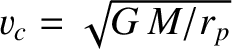, and that we wish to transfer it into a circular orbit of radius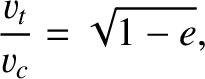, where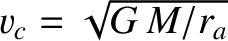. We can achieve this by temporarily placing the satellite in an elliptical orbit whose perihelion distance is, and whose aphelion distance is. It follows, from Equation (4.37), that the required eccentricity of the elliptical orbit is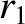(4.48)

According to Equation (4.46), we can transfer our satellite from its initial circular orbit into the temporary elliptical orbit by increasing its tangential velocity (by briefly switching on the satellite's rocket motor) by a factor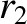(4.49)

We must next allow the satellite to execute half an orbit, so that it attains its aphelion distance, and then boost the tangential velocity by a factor [see Equation (4.47)]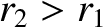(4.50)

The satellite will now be in a circular orbit at the aphelion distance,. This process is illustrated in Figure 4.4. Obviously, we can transfer our satellite from a larger to a smaller circular orbit by performing the preceding process in reverse. Note, finally, from Equation (4.46), that if we increase the tangential velocity of a satellite in a circular orbit about the Sun by a factor greater than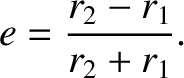then we will transfer it into a hyperbolic orbit (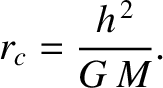), and it will eventually escape from the Sun's gravitational field.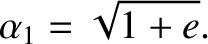Next: Elliptic orbits Up: Keplerian orbits Previous: Orbital energies
Richard Fitzpatrick 2016-03-31